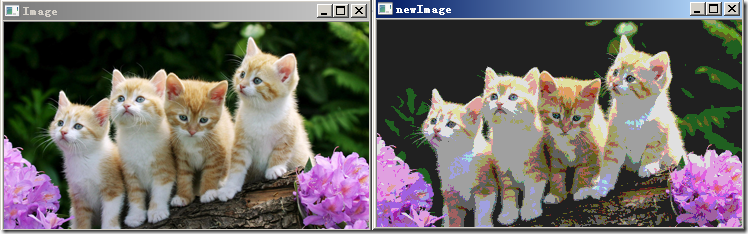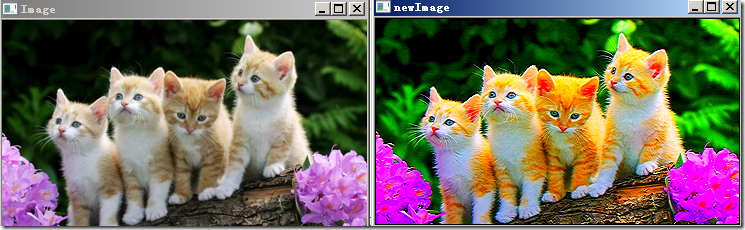opencv

OpenCV优化：图像的遍历4种方式

一、遍历图像的4种方式：at<typename>(i,j)

Mat类提供了一个at的方法用于取得图像上的点，它是一个模板函数，可以取到任何类型的图像上的点。下面我们通过一个图像处理中的实际来说明它的用法。1 void colorReduce(Mat& image,int div) 2 { 3     for(int i=0;i<image.rows;i++) 4     { 5         for(int j=0;j<image.cols;j++) 6         { 7             image.at<Vec3b>(i,j)=image.at<Vec3b>(i,j)/div*div+div/2; 8             image.at<Vec3b>(i,j)=image.at<Vec3b>(i,j)/div*div+div/2; 9             image.at<Vec3b>(i,j)=image.at<Vec3b>(i,j)/div*div+div/2;10         }11     }12 }image.at<uchar>(i,j)：取出灰度图像中i行j列的点。

image.at<Vec3b>(i,j)[k]：取出彩色图像中i行j列第k通道的颜色点。其中uchar,Vec3b都是图像像素值的类型，不要对Vec3b这种类型感觉害怕，其实在core里它是通过typedef Vec<T,N>来定义的，N代表元素的个数，T代表类型。

Mat_<uchar> im=image;

im(i,j)=im(i,j)/div*div+div/2;

二、高效一点：用指针来遍历图像1 void colorReduce(const Mat& image,Mat& outImage,int div) 2 { 3     // 创建与原图像等尺寸的图像 4     outImage.create(image.size(),image.type()); 5     int nr=image.rows; 6     // 将3通道转换为1通道 7     int nl=image.cols*image.channels(); 8     for(int k=0;k<nr;k++) 9     {10         // 每一行图像的指针11         const uchar* inData=image.ptr<uchar>(k);12         uchar* outData=outImage.ptr<uchar>(k);13         for(int i=0;i<nl;i++)14         {15             outData[i]=inData[i]/div*div+div/2;16         }17     }18 }三、更高效的方法1 void colorReduce(const Mat& image,Mat& outImage,int div) 2 { 3     int nr=image.rows; 4     int nc=image.cols; 5     outImage.create(image.size(),image.type()); 6     if(image.isContinuous()&&outImage.isContinuous()) 7     { 8         nr=1; 9         nc=nc*image.rows*image.channels();10     }11     for(int i=0;i<nr;i++)12     {13         const uchar* inData=image.ptr<uchar>(i);14         uchar* outData=outImage.ptr<uchar>(i);15         for(int j=0;j<nc;j++)16         {17             *outData++=*inData++/div*div+div/2;18         }19     }20 }image.step返回图像一行像素元素的个数（包括空白元素），image.elemSize()返回一个图像像素的大小。

&image.at<uchar>(i,j)=image.data+i*image.step+j*image.elemSize();

四、还有吗？用迭代器来遍历。

MatIterator_<Vec3b> it;

Mat_<Vec3b>::iterator it;

MatConstIterator<Vec3b> it; 或者

Mat_<Vec3b>::const_iterator it;1 void colorReduce(const Mat& image,Mat& outImage,int div) 2 { 3     outImage.create(image.size(),image.type()); 4     MatConstIterator_<Vec3b> it_in=image.begin<Vec3b>(); 5     MatConstIterator_<Vec3b> itend_in=image.end<Vec3b>(); 6     MatIterator_<Vec3b> it_out=outImage.begin<Vec3b>(); 7     MatIterator_<Vec3b> itend_out=outImage.end<Vec3b>(); 8     while(it_in!=itend_in) 9     {10         (*it_out)=(*it_in)/div*div+div/2;11         (*it_out)=(*it_in)/div*div+div/2;12         (*it_out)=(*it_in)/div*div+div/2;13         it_in++;14         it_out++;15     }16 }五、图像的邻域操作

)-image(i,j-1)-image(i,j+1)。1 void ImgFilter2d(const Mat &image,Mat& result) 2 { 3     result.create(image.size(),image.type()); 4     int nr=image.rows; 5     int nc=image.cols*image.channels(); 6     for(int i=1;i<nr-1;i++) 7     { 8         const uchar* up_line=image.ptr<uchar>(i-1);//指向上一行 9         const uchar* mid_line=image.ptr<uchar>(i);//当前行10         const uchar* down_line=image.ptr<uchar>(i+1);//下一行11         uchar* cur_line=result.ptr<uchar>(i);12         for(int j=1;j<nc-1;j++)13         {14             cur_line[j]=saturate_cast<uchar>(5*mid_line[j]-mid_line[j-1]-mid_line[j+1]-15                 up_line[j]-down_line[j]);16         }17     }18     // 把图像边缘像素设置为019     result.row(0).setTo(Scalar(0));20     result.row(result.rows-1).setTo(Scalar(0));21     result.col(0).setTo(Scalar(0));22     result.col(result.cols-1).setTo(Scalar(0));23 }1，staturate_cast<typename>是一个类型转换函数，程序里是为了确保运算结果还在uchar范围内。

2，row和col方法返回图像中的某些行或列，返回值是一个Mat。

3，setTo方法将Mat对像中的点设置为一个值，Scalar(n)为一个灰度值，Scalar(a,b,c)为一个彩色值。

六、图像的算术运算

Mat类把很多算数操作符都进行了重载，让它们来符合矩阵的一些运算，如果+、-、点乘等。

1 vector<Mat> planes;2 // 将image分为三个通道图像存储在planes中3 split(image,planes);4 planes+=image2;5 // 将planes中三幅图像合为一个三通道图像6 merge(planes,result);

2017/09/06
0
0

选自Medium 　　作者：Vladimir Goncharov 　　机器之心编译 　　参与：Huiyuan Zhuo、思源、刘晓坤 　　 　　作者 Vladimir Goncharov 平常主要关注与研究两个主题：PHP 和 Server Adm...

2018/06/25
0
0
python 图像处理：一福变五福

crossin
01/31
60
0
Python各类图像库的图片读写方式总结

chenxueying1993
2018/04/24
0
0

OpenCV是Intel资助的开源计算机视觉库。它由一系列 C 函数和少量 C++ 类构成，实现了图像处理和计算机视觉方面的很多通用算法。 OpenCV 拥有包括 300 多个C/C++函数的跨平台的中、高层 API。...

2008/12/10
3.3K
3

arduino项目-1. 模拟楼道灯

@toc 1.1 情景说明 说明 漆黑的夜晚，当有人非法进入一所房屋，房屋内的灯在恰当的时间亮起，也许会有效阻止非法活动的继续。 效果展示 1.2 实验器材 器材名称 数量 继电器 1 人体红外感应器...

acktomas
30分钟前
4
0
Nacos 常见问题及解决方法

Nacos 开源至今已有一年，在这一年里，得到了很多用户的支持和反馈。在与社区的交流中，我们发现有一些问题出现的频率比较高，为了能够让用户更快的解决问题，我们总结了这篇常见问题及解决方...

36分钟前
6
0
pinyin4j 满足中文转拼音的需求

edison_kwok
41分钟前
5
0

Substrate框架将区块链的众多功能都模块化，对于开发者来说，只是一个选择的问题，同时还保持了众多的可以定制的功能和模块，比如底层通信模块，比如账户体系，比如共识机制等都是可以自己定...

IPSE
47分钟前
156
0